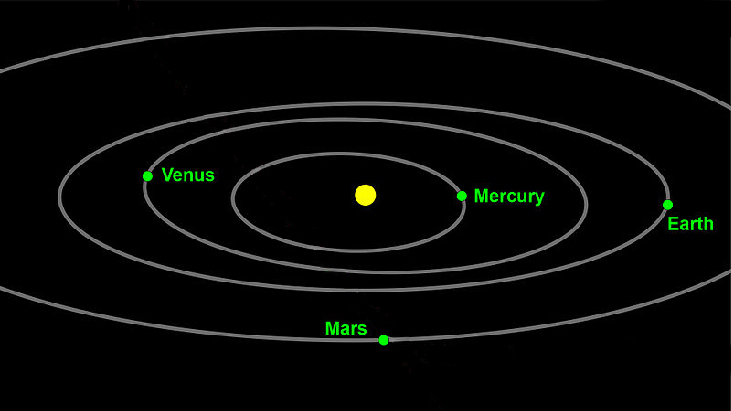# 10.4 Polar coordinates: graphs

 Page 1 / 16
In this section you will:
• Test polar equations for symmetry.
• Graph polar equations by plotting points.

The planets move through space in elliptical, periodic orbits about the sun, as shown in [link] . They are in constant motion, so fixing an exact position of any planet is valid only for a moment. In other words, we can fix only a planet’s instantaneous position. This is one application of polar coordinates    , represented as $\text{\hspace{0.17em}}\left(r,\theta \right).\text{\hspace{0.17em}}$ We interpret $\text{\hspace{0.17em}}r\text{\hspace{0.17em}}$ as the distance from the sun and $\text{\hspace{0.17em}}\theta \text{\hspace{0.17em}}$ as the planet’s angular bearing, or its direction from a fixed point on the sun. In this section, we will focus on the polar system and the graphs that are generated directly from polar coordinates.Planets follow elliptical paths as they orbit around the Sun. (credit: modification of work by NASA/JPL-Caltech)

## Testing polar equations for symmetry

Just as a rectangular equation such as $\text{\hspace{0.17em}}y={x}^{2}\text{\hspace{0.17em}}$ describes the relationship between $\text{\hspace{0.17em}}x\text{\hspace{0.17em}}$ and $\text{\hspace{0.17em}}y\text{\hspace{0.17em}}$ on a Cartesian grid, a polar equation describes a relationship between $\text{\hspace{0.17em}}r\text{\hspace{0.17em}}$ and $\text{\hspace{0.17em}}\theta \text{\hspace{0.17em}}$ on a polar grid. Recall that the coordinate pair $\text{\hspace{0.17em}}\left(r,\theta \right)\text{\hspace{0.17em}}$ indicates that we move counterclockwise from the polar axis (positive x -axis) by an angle of $\text{\hspace{0.17em}}\theta ,\text{\hspace{0.17em}}$ and extend a ray from the pole (origin) $\text{\hspace{0.17em}}r\text{\hspace{0.17em}}$ units in the direction of $\text{\hspace{0.17em}}\theta .\text{\hspace{0.17em}}$ All points that satisfy the polar equation are on the graph.

Symmetry is a property that helps us recognize and plot the graph of any equation. If an equation has a graph that is symmetric with respect to an axis, it means that if we folded the graph in half over that axis, the portion of the graph on one side would coincide with the portion on the other side. By performing three tests, we will see how to apply the properties of symmetry to polar equations. Further, we will use symmetry (in addition to plotting key points, zeros, and maximums of $\text{\hspace{0.17em}}r\right)\text{\hspace{0.17em}}$ to determine the graph of a polar equation.

In the first test, we consider symmetry with respect to the line $\text{\hspace{0.17em}}\theta =\frac{\pi }{2}\text{\hspace{0.17em}}$ ( y -axis). We replace $\text{\hspace{0.17em}}\left(r,\theta \right)\text{\hspace{0.17em}}$ with $\text{\hspace{0.17em}}\left(-r,-\theta \right)\text{\hspace{0.17em}}$ to determine if the new equation is equivalent to the original equation. For example, suppose we are given the equation $\text{\hspace{0.17em}}r=2\mathrm{sin}\text{\hspace{0.17em}}\theta ;$

This equation exhibits symmetry with respect to the line $\text{\hspace{0.17em}}\theta =\frac{\pi }{2}.$

In the second test, we consider symmetry with respect to the polar axis ( $\text{\hspace{0.17em}}x$ -axis). We replace $\text{\hspace{0.17em}}\left(r,\theta \right)\text{\hspace{0.17em}}$ with $\text{\hspace{0.17em}}\left(r,-\theta \right)\text{\hspace{0.17em}}$ or $\text{\hspace{0.17em}}\left(-r,\pi -\theta \right)\text{\hspace{0.17em}}$ to determine equivalency between the tested equation and the original. For example, suppose we are given the equation $\text{\hspace{0.17em}}r=1-2\mathrm{cos}\text{\hspace{0.17em}}\theta .$

The graph of this equation exhibits symmetry with respect to the polar axis.

In the third test, we consider symmetry with respect to the pole (origin). We replace $\text{\hspace{0.17em}}\left(r,\theta \right)\text{\hspace{0.17em}}$ with $\text{\hspace{0.17em}}\left(-r,\theta \right)\text{\hspace{0.17em}}$ to determine if the tested equation is equivalent to the original equation. For example, suppose we are given the equation $\text{\hspace{0.17em}}r=2\mathrm{sin}\left(3\theta \right).$

$\begin{array}{c}\text{\hspace{0.17em}}\text{\hspace{0.17em}}\text{\hspace{0.17em}}\text{\hspace{0.17em}}\text{\hspace{0.17em}}r=2\mathrm{sin}\left(3\theta \right)\\ -r=2\mathrm{sin}\left(3\theta \right)\end{array}$

The equation has failed the symmetry test , but that does not mean that it is not symmetric with respect to the pole. Passing one or more of the symmetry tests verifies that symmetry will be exhibited in a graph. However, failing the symmetry tests does not necessarily indicate that a graph will not be symmetric about the line $\text{\hspace{0.17em}}\theta =\frac{\pi }{2},\text{\hspace{0.17em}}$ the polar axis, or the pole. In these instances, we can confirm that symmetry exists by plotting reflecting points across the apparent axis of symmetry or the pole. Testing for symmetry is a technique that simplifies the graphing of polar equations, but its application is not perfect.

what is the function of sine with respect of cosine , graphically
tangent bruh
Steve
cosx.cos2x.cos4x.cos8x
sinx sin2x is linearly dependent
what is a reciprocal
The reciprocal of a number is 1 divided by a number. eg the reciprocal of 10 is 1/10 which is 0.1
Shemmy
Reciprocal is a pair of numbers that, when multiplied together, equal to 1. Example; the reciprocal of 3 is ⅓, because 3 multiplied by ⅓ is equal to 1
Jeza
each term in a sequence below is five times the previous term what is the eighth term in the sequence
I don't understand how radicals works pls
How look for the general solution of a trig function
stock therom F=(x2+y2) i-2xy J jaha x=a y=o y=b
sinx sin2x is linearly dependent
cr
root under 3-root under 2 by 5 y square
The sum of the first n terms of a certain series is 2^n-1, Show that , this series is Geometric and Find the formula of the n^th
cosA\1+sinA=secA-tanA
Wrong question
why two x + seven is equal to nineteen.
The numbers cannot be combined with the x
Othman
2x + 7 =19
humberto
2x +7=19. 2x=19 - 7 2x=12 x=6
Yvonne
because x is 6
SAIDI
what is the best practice that will address the issue on this topic? anyone who can help me. i'm working on my action research.
simplify each radical by removing as many factors as possible (a) √75
how is infinity bidder from undefined?ByBy OpenStaxBy OpenStaxBy Madison ChristianByBy Janet ForresterBy Brooke DelaneyBy Robert MurphyBy Richley CrapoBy OpenStaxBy Tony Pizur